• Kmeans文本聚类
2021-05-21 07:00:59

1、数据准备：

文本按行存储，分词以空格分割；

2、embedding：

使用word2vec计算每个词组的embedding；

./word2vec -train src.txt -output dst.vec -cbow 0 -size 200 -window 5 -negative 0 -hs 1 -sample 1e-3 -threads 2 -binary 0

./word2vec -train src.txt -output dst.vec -cbow 0 -size 200 -window 5 -negative 0 -hs 1 -sample 1e-3 -threads 2 -binary 1

3、IDF计算：

TF-IDF = 词频(TF) x 逆向文档频率(IDF)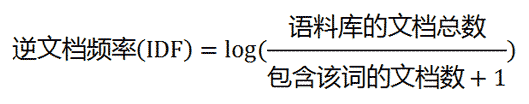4、样本归一：

计算文章标题向量所有元素之和；

文章标题向量每个元素除以向量和；

结果写入新建文章标题向量；

5、构建样本：

计算每个单词(汉字)归一值与相似度TOP(n)的乘积，生成n个元素的向量；

将每个单词(汉字)的向量元素逐一相加求和，生成该文章标题对应的向量；

6、训练模型：

def do_texts_kmeans(data):

'''训练Kmeans模型'''

kmeans = KMeans(n_clusters=zyb_cluster, max_iter=zyb_maxiter)

kmeans.fit(data)

return kmeans

7、保存模型：

def do_kmeans_model(kmeans):

'''保存Kmeans模型'''

pickle.dump(kmeans, open(file, "wb"))

8、模型预测：

def do_texts_predict(data):

'''预测Kmeans模型'''

return [kmeans.predict([line]) for line in data]

9、主体流程：

构建分词矩阵 -> 计算单词词频 -> 构建分词字典 -> 构建文档向量 -> 生成TFIDF结果 -> 生成归一化结果 -> 构建训练数据 -> 生成Kmeans模型 -> 输出Kmeans模型

更多相关内容
• ## K-Means聚类算法的实现（C语言）

万次阅读 多人点赞 2014-11-14 23:07:44
最近做了聚类实验——K-means算法

最近做了聚类实验，就写了下K-Means算法，C语言实现.

实验给出的数据集比较小,总共有11个：(2, 10), (2, 5), (8, 4), (5, 8), (7, 5), (6, 4), (1, 2), (4, 9), (7, 3), (1, 3), (3, 9)

代码运行的聚类结果：

Cluster-1： (2, 10),  (5, 8),  (4, 9),  (3, 9)

Cluster-2： (8, 4),  (7, 5),  (6, 4),  (7, 3)

Cluster-3： (2, 5),  (1, 2),  (1, 3)

Clementine软件运行结果：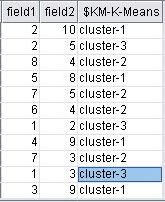可以看出与软件运行结果相比代码是正确的.

Code：

#include <stdio.h>
#include <string.h>
#include <stdlib.h>
#include <math.h>
#include <time.h>

#define N 11
#define K 3

typedef struct
{
float x;
float y;
}Point;

int center[N];  ///  判断每个点属于哪个簇

Point point[N] = {
{2.0, 10.0},
{2.0, 5.0},
{8.0, 4.0},
{5.0, 8.0},
{7.0, 5.0},
{6.0, 4.0},
{1.0, 2.0},
{4.0, 9.0},
{7.0, 3.0},
{1.0, 3.0},
{3.0, 9.0}
};

Point mean[K];  ///  保存每个簇的中心点

float getDistance(Point point1, Point point2)
{
float d;
d = sqrt((point1.x - point2.x) * (point1.x - point2.x) + (point1.y - point2.y) * (point1.y - point2.y));
return d;
}

/// 计算每个簇的中心点
void getMean(int center[N])
{
Point tep;
int i, j, count = 0;
for(i = 0; i < K; ++i)
{
count = 0;
tep.x = 0.0;   /// 每算出一个簇的中心点值后清0
tep.y = 0.0;
for(j = 0; j < N; ++j)
{
if(i == center[j])
{
count++;
tep.x += point[j].x;
tep.y += point[j].y;
}
}
tep.x /= count;
tep.y /= count;
mean[i] = tep;
}
for(i = 0; i < K; ++i)
{
printf("The new center point of %d is : \t( %f, %f )\n", i+1, mean[i].x, mean[i].y);
}
}

/// 计算平方误差函数
float getE()
{
int i, j;
float cnt = 0.0, sum = 0.0;
for(i = 0; i < K; ++i)
{
for(j = 0; j < N; ++j)
{
if(i == center[j])
{
cnt = (point[j].x - mean[i].x) * (point[j].x - mean[i].x) + (point[j].y - mean[i].y) * (point[j].y - mean[i].y);
sum += cnt;
}
}
}
return sum;
}

/// 把N个点聚类
void cluster()
{
int i, j, q;
float min;
float distance[N][K];
for(i = 0; i < N; ++i)
{
min = 999999.0;
for(j = 0; j < K; ++j)
{
distance[i][j] = getDistance(point[i], mean[j]);

/// printf("%f\n", distance[i][j]);  /// 可以用来测试对于每个点与3个中心点之间的距离
}
for(q = 0; q < K; ++q)
{
if(distance[i][q] < min)
{
min = distance[i][q];
center[i] = q;
}
}
printf("( %.0f, %.0f )\t in cluster-%d\n", point[i].x, point[i].y, center[i] + 1);
}
printf("-----------------------------\n");
}

int main()
{
int i, j, n = 0;
float temp1;
float temp2, t;
printf("----------Data sets----------\n");
for(i = 0; i < N; ++i)
{
printf("\t( %.0f, %.0f )\n", point[i].x, point[i].y);
}
printf("-----------------------------\n");

/*
可以选择当前时间为随机数
srand((unsigned int)time(NULL));

for(i = 0; i < K; ++i)
{
j = rand() % K;
mean[i].x = point[j].x;
mean[i].y = point[j].y;
}
*/
mean.x = point.x;      /// 初始化k个中心点
mean.y = point.y;

mean.x = point.x;
mean.y = point.y;

mean.x = point.x;
mean.y = point.y;

cluster();          /// 第一次根据预设的k个点进行聚类
temp1 = getE();        ///  第一次平方误差
n++;                   ///  n计算形成最终的簇用了多少次

printf("The E1 is: %f\n\n", temp1);

getMean(center);
cluster();
temp2 = getE();        ///  根据簇形成新的中心点，并计算出平方误差
n++;

printf("The E2 is: %f\n\n", temp2);

while(fabs(temp2 - temp1) != 0)   ///  比较两次平方误差 判断是否相等，不相等继续迭代
{
temp1 = temp2;
getMean(center);
cluster();
temp2 = getE();
n++;
printf("The E%d is: %f\n", n, temp2);
}

printf("The total number of cluster is: %d\n\n", n);  /// 统计出迭代次数
system("pause");
return 0;
}


展开全文• 原标题：r语言文本分析-主题模型-文本分类-文本聚类 数据介绍从凤凰新闻采集：财经、军事、科技、禅道四类文章总共207篇。如下： 文本数据处理文本分析最关键就是分词了，只有分好了词才能去寻找关键特征，最后根据...

原标题：r语言文本分析-主题模型-文本分类-文本聚类数据介绍

从凤凰新闻采集：财经、军事、科技、禅道四类文章总共207篇。

如下：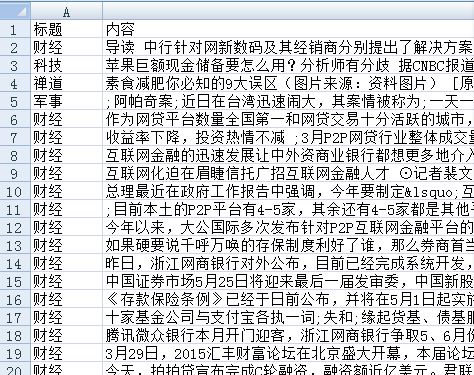文本数据处理

文本分析最关键就是分词了，只有分好了词才能去寻找关键特征，最后根据自己的需要做一些分析工作，我比较喜欢结巴分词(比较稳定)。以前用过李建的rwordseg，后来由于rjava不稳定，加载不了自定义字典，另一个原因是结巴安装比较简单，尤其对我这样非it专业出身的人来说省了不少事情。

setwd("e://r语言学习//r代码")

data

######################数字处理##########################

removeNumbers = function(x) { ret = gsub("[0-9０１２３４５６７８９]","",x) }

sample.words

doc=c(NULL)

for(i in 1:dim(data)){

doc=c(doc,sample.words[i])

}

#################################################################

# 字母处理

doc=gsub(pattern="[a-zA-Z]+","",doc)

#################################################################

#分词

library(jiebaR)

tagger

doc_CN=list()

for(j in 1:length(doc)){

doc_CN[[j]]=c( tagger<=doc[j])

}

mystopwords

###################去停用词##############################

sample.words

for(i in 1:length(sample.words))

{

sample.words[[i]]=sample.words[[i]][!sample.words[[i]]%in%mystopwords]

sample.words[[i]]=subset(sample.words[[i]], nchar(as.character(sample.words[[i]]))>1 )

sample.words[[i]]=paste(sample.words[[i]],sep = " ",collapse =" ")

}

#######################################################

主题模型训练

主题模型，太他妈的理论了。足以证明学数学好的也没什么了不起，还是看不懂。也许是自己学艺不精。就简单介绍一下吧。主题模型属于机器学习里面的无监督学习类的模型，我想大家肯定想到了kmeans 聚类，kmeans是从文档层面的聚类，而主题模型是从文档内部的主题去聚类。kmeans是你给定类的个数，机器自动聚成多少类，而主题模型就是你给定主题个数，机器自动聚成多少主题。好了，其他的不说了，再说你就晕了，会用就行。

############################################

library(lda)

corpus

num.topics

## Initialize the params

params

poliblog.ratings

result

K=num.topics,

vocab=corpus$vocab, num.e.iterations=30, num.m.iterations=12, alpha=1.0, eta=0.1, poliblog.ratings / 100, params, variance=0.25, lambda=1.0, logistic=FALSE, method="sLDA") ## Make a pretty picture. Topics 2, paste, collapse=" ") aa=length(Topics) t=c() for(i in 1:aa) {t[i]=paste(i,Topics[i],sep="")} a=apply(result$document_sums,

1,sum)

names(a)

p=data.frame(a=t,b=a)

p=p[order(p[,2],decreasing=T),]

a1=c()

c=c("a","b","c","d","e","f","g","h","i","j","k","l","m","n","o","p","q","r","s","t","u","v","w","x","y","z"

,"za","zb","zc","zd")

for(i in 1:aa)

{

a1[i]= paste(c[i],p$a[i],sep="") } p1=data.frame(a=a1,主题得分=p$b)

library(ggplot2)

ggplot(data=p1, aes(x=a, y=主题得分, fill=主题得分)) +

geom_bar(colour="black", stat="identity") +

labs(x = "主题", y = "得分") + ggtitle("文档主题排名顺序")+ coord_flip()

Topics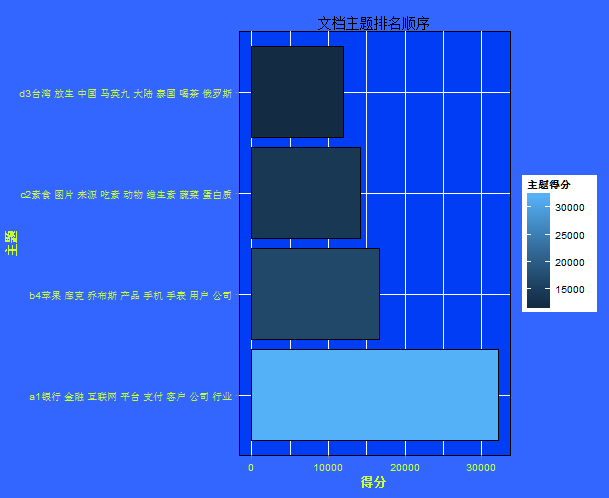a=c()

b=c()

for(i in 1:5)

{

a=c(a,Topics[,i])

b=c(b,rep(paste("主题",i,sep=""),20))

}

a = table(a, b)

a = as.matrix(a)

library(wordcloud)

comparison.cloud(a, scale = c(1, 1.5), rot.per = 0.5, colors = brewer.pal(ncol(a), "Dark2"))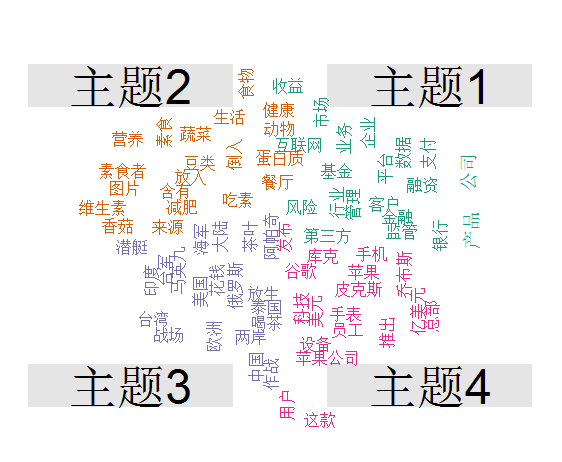主题1：金融 主题2 ：禅道 主题3 ：军事 主题4 科技

文本分类

提到文本聚类就要说到r语言的tm包，该包的作用就是生成文本文档矩阵，这个比较方便。但是如果你的文本特征不是词频，tf-IDF，那么他们包就没什么用了。

library("tm")

reuters =VCorpus(VectorSource(doc_CN))

reuters

data_stw

reuters=tm_map(reuters,removeWords,data_stw)

# 删除停用词

############################

####生成tfidf特征##################

control=list(removePunctuation=T,minDocFreq=5,wordLengths = c(1, Inf),weighting = weightTfIdf)

doc.tdm=TermDocumentMatrix(reuters,control)

length(doc.tdm$dimnames$Terms)

tdm_removed=removeSparseTerms(doc.tdm, 0.97)

length(tdm_removed$dimnames$Terms)

mat = as.matrix(tdm_removed)####转换成文档矩阵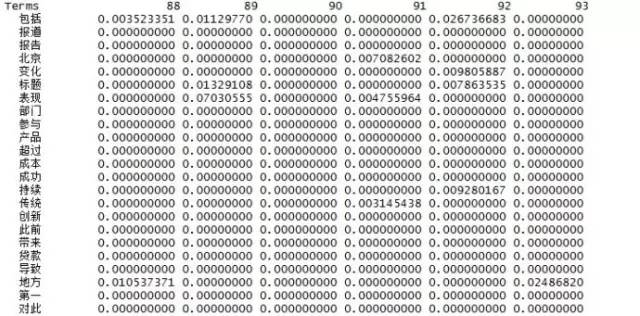classifier = naiveBayes(mat[1:x,], as.factor(data$标题[1:x]) )##贝叶斯分类器，训练 predicted = predict(classifier, mat[z:y,]);#预测 A=table(data$标题[z:y], predicted)#预测交叉矩阵

predicted财经 禅道 军事 科技

财经 10 28 34 1 禅道 0 41 4 0 军事 0 10 25 0 科技 4 21 18 11

b1=length(which(predicted==data$标题[z:y]))/length(predicted)#计算召回率 > b1 0.4202899##有点低哈！！！！ ###寻找其他的机器学习分类算法 library(RTextTools) container = create_container(mat[1:y,], as.factor(data$标题[1:y]) ,

trainSize=1:x, testSize=1:y,virgin=TRUE)

models = train_models(container, algorithms=c("BAGGING" , "MAXENT" , "NNET" , "RF" , "SVM" , "TREE" ))

results = classify_models(container, models)

# recall accuracy

森林=recall_accuracy(as.numeric(as.factor(data$标题[z:y])), results[,"FORESTS_LABEL"]) 最大熵=recall_accuracy(as.numeric(as.factor(data$标题[z:y])), results[,"MAXENTROPY_LABEL"])

决策树=recall_accuracy(as.numeric(as.factor(data$标题[z:y])), results[,"TREE_LABEL"]) 袋袋=recall_accuracy(as.numeric(as.factor(data$标题[z:y])), results[,"BAGGING_LABEL"])

向量机=recall_accuracy(as.numeric(as.factor(data$标题[z:y])), results[,"SVM_LABEL"]) 神经网络=recall_accuracy(as.numeric(as.factor(data$标题[z:y])), results[,"NNETWORK_LABEL"])

a=c()

c=c()

e=c()

a=cbind( 随机森林=as.vector(results[,"FORESTS_LABEL"]), 决策树=as.vector(results[,"TREE_LABEL"]) , 支持向量机=as.vector(results[,"SVM_LABEL"]),

贝叶斯=as.vector(predicted), 最大熵=as.vector(results[,"MAXENTROPY_LABEL"]),袋袋=as.vector(results[,"BAGGING_LABEL"]),

神经网络=as.vector( results[,"NNETWORK_LABEL"]))

for(i in 1:length(results[,"FORESTS_LABEL"][z:y]))

{

b=table(a[i,])

c[i]

}

模型预测=cbind(a,组合模型=c)

A=table(data$标题[z:y],c) b=length(which(c==data$标题[z:y]))/length(c)

组合模型=b

e=c(贝叶斯=b1,森林=森林,最大熵=最大熵,决策树=决策树,袋袋=袋袋,向量机=向量机,神经网络=神经网络,组合投票=组合模型)

##结果该满意了吧！！！

> e 贝叶斯 森林 最大熵 决策树 袋袋 向量机 神经网络 组合投票 0.4202899 1.0000000 1.0000000 0.5893720 1.0000000 0.3526570 0.9033816 1.0000000

文本聚类

文本聚类就没什么技术含量了，主要原因是其实非监督学习，效果一般不是很好。

data=t(mat[,1:50])

data.scale

d

fit

plot(fit,main="文本聚类")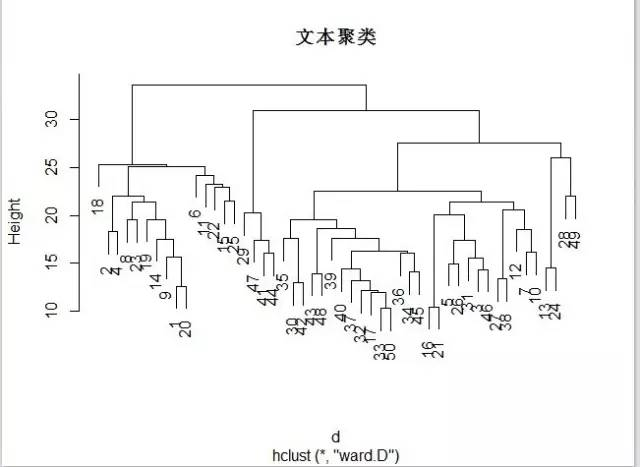来源：l000NGag0的博客

责任编辑：

展开全文• 文本聚类正所谓人以类聚，物以群分。人类获取并积累信息时常常需要整理数据，将相似的数据归档到一起。许多数据分析需求都归结为自动发现大量样本之间的相似性，并将其划分为不同的小组，这种根据相似性归档的任务...

文本聚类

正所谓人以类聚，物以群分。人类获取并积累信息时常常需要整理数据，将相似的数据归档到一起。许多数据分析需求都归结为自动发现大量样本之间的相似性，并将其划分为不同的小组，这种根据相似性归档的任务称为聚类。

基本概念

聚类(cluster analysis)指的是将给定对象的集合划分为不同子集的过程，目标是使得每个子集内部的元素尽量相似，不同子集间的元素尽量不相似。这些子集又被称为簇(cluster)，一般没有交集。聚类的概念如图所示。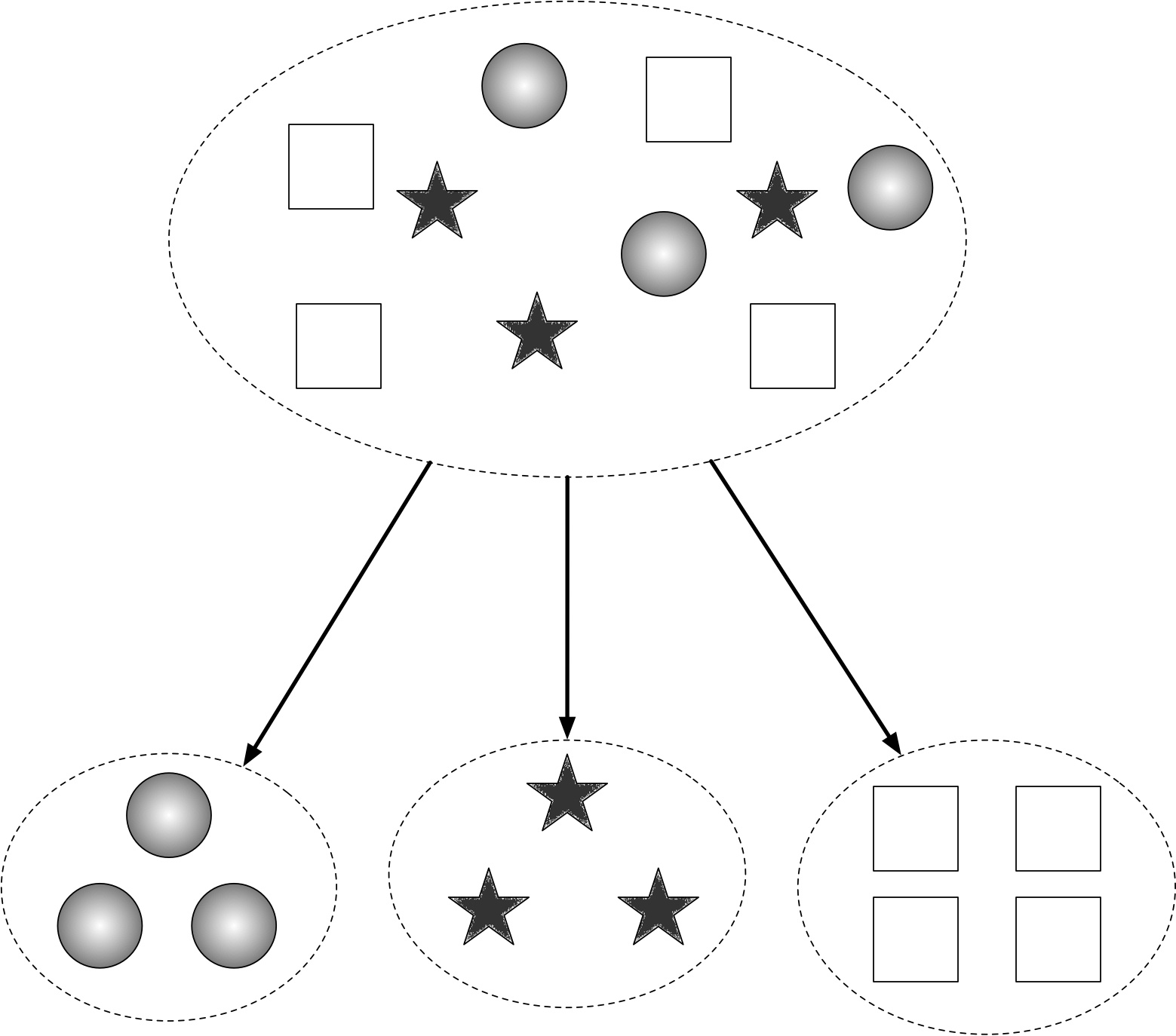聚类

文本聚类(document clustering)指的是对文档进行的聚类，被广泛用于文本挖掘和信息检索领域。最初文本聚类仅用于文本归档，后来人们又挖掘出了许多新用途。比如改善搜索结果，生成同义词等等。

在文本的预处理中，聚类同样可以发挥作用。比如在标注语料之前，通常需要从生语料中选取一定数量有代表性的文档作为样本。假设需要标注N篇，则可以将这些生语料聚类为N个簇，每个簇随机选取一篇即可。利用每个簇内元素都是相似的这个性质，聚类甚至可以用于文本排重。

目前市面上常见的聚类算法是k-means，但HanLP不光实现了k-means，还实现了速度更快效果更好的repeated bisection算法。我们将在文末比较两种算法的速度与准确率。

文本聚类模块

在HanLP中，聚类算法实现为ClusterAnalyzer，用户可以将其想象为一个文档id到文档向量的映射容器。创建ClusterAnalyzer对象后，向其中加入若干文档之后即可调用k-means接口得到指定数量的簇。文档id在实现上是泛型的，Java用户可以将文档String标题，或数据库Integer主键作为id的类型。

此处以某音乐网站中的用户聚类为案例讲解聚类模块的用法。假设该音乐网站将6位用户点播的歌曲的流派记录下来，并且分别拼接为6段文本。给定用户名称与这6 段播放历史，要求将这6名用户划分为3个簇。

首先，我们需要创建ClusterAnalyzer对象，并向其加入文档。Java示例如下：

ClusterAnalyzer analyzer = new ClusterAnalyzer();

analyzer.addDocument("赵一", "流行, 流行, 流行, 流行, 流行, 流行, 流行, 流行, 流行, 流行, 蓝调, 蓝调, 蓝调, 蓝调, 蓝调, 蓝调, 摇滚, 摇滚, 摇滚, 摇滚");

analyzer.addDocument("钱二", "爵士, 爵士, 爵士, 爵士, 爵士, 爵士, 爵士, 爵士, 舞曲, 舞曲, 舞曲, 舞曲, 舞曲, 舞曲, 舞曲, 舞曲, 舞曲");

analyzer.addDocument("张三", "古典, 古典, 古典, 古典, 民谣, 民谣, 民谣, 民谣");

analyzer.addDocument("李四", "爵士, 爵士, 爵士, 爵士, 爵士, 爵士, 爵士, 爵士, 爵士, 金属, 金属, 舞曲, 舞曲, 舞曲, 舞曲, 舞曲, 舞曲");

analyzer.addDocument("王五", "流行, 流行, 流行, 流行, 摇滚, 摇滚, 摇滚, 嘻哈, 嘻哈, 嘻哈");

analyzer.addDocument("马六", "古典, 古典, 古典, 古典, 古典, 古典, 古典, 古典, 摇滚");

文档加入后，ClusterAnalyzer内部会自动对其分词、去除停用词、转换为词袋向量，如表1所示。

流行

蓝调

摇滚

爵士

舞曲

古典

民谣

金属

嘻哈

赵一

10

6

4

0

0

0

0

0

0

钱二

0

0

0

8

9

0

0

0

0

张三

0

0

0

0

0

4

4

0

0

李四

0

0

0

9

6

0

0

2

0

王五

4

0

3

0

0

0

0

0

3

马六

0

0

1

0

0

8

0

0

0

文本聚类中的词袋向量

有了这些向量后，只需调用ClusterAnalyzer的kmeans或repeatedBisection方法就可以得到指定数量的簇，以3为例：

System.out.println(analyzer.kmeans(3));

System.out.println(analyzer.repeatedBisection(3));

该方法返回指定数量的簇构成的集合，每个簇是一个Set，内部元素为文档的id。此处由于id是姓名，所以可以打印出来直观地感受效果：

[[李四, 钱二], [王五, 赵一], [张三, 马六]]

根据该结果，李四和钱二同属一个簇。对照表1，这二人都喜欢爵士和舞曲。类似地，王五和赵一都喜欢流行和摇滚音乐；张三和马六都喜欢古典音乐。通过k-means聚类算法，我们成功地将用户按兴趣分组，得到了“人以群分”的效果。

聚类结果中簇的顺序是随机的，每个簇中的元素也是无序的。由于k-means是个随机算法，有小概率得到不同的结果。

该聚类模块可以接受任意文本作为文档，而不需要用特殊分隔符隔开单词。另外，该模块还接受单词列表作为输入，用户可以将英文、日文等预先切分为单词列表后输入本模块。统计方法适用于所有语种，不必拘泥于中文。

自动判断聚类个数k

通过上面的介绍，用户可能觉得聚类个数k这个超参数很难准确指定。在repeated bisection算法中，有一种变通的方法，那就是通过给准则函数的增幅设定阈值beta来自动判断k。此时算法的停机条件为，当一个簇的二分增幅小于beta时不再对该簇进行划分，即认为这个簇已经达到最终状态，不可再分；当所有簇都不可再分时，算法终止，此时产生的聚类数量就不再需要人工指定了。

在HanLP中，repeated bisection算法提供了3种接口，分别需要指定k、beta或两者同时指定。当同时指定k和beta时，满足两者的停止条件中任意一个算法都会停止。当只指定一个时，另一个停止条件不起作用。这三个接口列举如下：

public List> repeatedBisection(int nclusters)

public List> repeatedBisection(double limit_eval)

public List> repeatedBisection(int nclusters, double limit_eval)

对于上一个例子，以beta=1.0作为参数试试自动判断聚类个数k，发现恰好可以得到理想的结果，Java示例：

System.out.println(analyzer.repeatedBisection(1.0)); // 自动判断聚类数量k

标准化评测

前面介绍的音乐案例只有6个样本，只能说是玩具数据(toy data)。用玩具数据来调试算法很方便，但不足以说明算法的实用性。本节我们将介绍聚类任务的标准化评测手段，并且给出两种算法的分值。

聚类任务常用的一种评测手段是沿用分类任务的F1值，将一些人工分好类别的文档去掉标签交给聚类分析器，统计结果中有多少同类别的文档属于同一个簇。

语料库

本次评测选择搜狗实验室提供的文本分类语料的一个子集，笔者称其为“搜狗文本分类语料库迷你版”。该迷你版语料库分为5个类目，每个类目下1000篇文章，共计5000篇文章。配套代码将自动下载该语料到data/test/搜狗文本分类语料库迷你版，其目录结构如下所示：

搜狗文本分类语料库迷你版

├── 体育

│ └── 1.txt

│ └── 2.txt

│ └── 3.txt

│ └── ...

├── 健康

│ └── ...

├── 军事

│ └── ...

├── 教育

│ └── ...

└── 汽车

└── ...

评测试验

评测程序遍历子目录读取文档，以子目录+文件名作为id将文档传入聚类分析器进行聚类，并且计算F1值返回。该计算过程封装为接口com.hankcs.hanlp.mining.cluster.ClusterAnalyzer#evaluate，欢迎用户自行查阅。此处仅演示评测接口的调用，Java用户可参考com.hankcs.demo.DemoTextClusteringFMeasure：

for (String algorithm : new String[]{"kmeans", "repeated bisection"})

{

System.out.printf("%s F1=%.2f\n", algorithm, ClusterAnalyzer.evaluate(CORPUS_FOLDER, algorithm) * 100);

}

两者的输出汇总如表2所示。

F1

耗时

kmeans

83.74

67秒

repeated bisection

85.58

24秒

对比两种算法，repeated bisection不仅准确率比kmeans更高，而且速度是kmeans的三倍。然而repeated bisection成绩波动较大，需要多运行几次才可能得出这样的结果。也许85%左右的准确率并不好看，但考虑到聚类是一种无监督学习，其性价比依然非常可观。

参考文献

Steinbach M, Karypis G, Kumar V. A comparison of document clustering techniques[C]//KDD workshop on text mining. 2000, 400(1): 525-526.

展开全文• K-means算法是很典型的基于距离的聚类算法，采用距离作为相似性的评价指标，即认为两个对象的距离越近，其相似度就越大。该算法认为簇是由距离靠近的对象组成的，因此把得到紧凑且独立的簇作为最终目标。算法过程...
• 最短距离聚类，有关资料最短距离聚类，有关资料最短距离聚类，有关资料
• Affinity Propagation (AP)聚类是2007年在Science杂志上提出的一种新的聚类算法。它根据N个数据点之间的相似度进行聚类,这些相似度可以是对称的,即两个数据点互相之间的相似度一样(如欧氏距离);也可以是不对称的,即...
• word2vec使用说明及源码介绍 1.下载地址 2....关键词聚类 -train Result_Country.txt 表示的是输入文件是Result_Country.txt -output vectors.bin 输出文件是vectors.bin 基础性文章，希望对你有所帮助~word2vec 词向量 相似度
• c语言实现的传统k_meams算法可以实现对任意维数的数据进行统计，计算中心点，并进行分类数据以文本的形式读入，以文本的形式输出。
• 最近做了一个数据挖掘的项目，挖掘过程中用到了K-means聚类方法，但是由于根据行业经验确定的聚类数过多并且并不一定是我们获取到数据的真实聚类数，所以，我们希望能从数据自身出发去确定真实的聚类数，也就是对...机器学习
• k-means算法 算法思想 K-means主要思想是在给定K值和若干样本（点）的情况下，把每个样本（点）分到离其最近的类簇中心点所代表的类簇中，所有点分配完毕...用C语言实现 自定义的数据集共有15个： (2, 7) (2, 5) ...
• ## 聚类

千次阅读 2013-08-16 15:19:20
现在有一些数据需要做聚类处理。  那什么叫做聚类呢 跟分类有什么区别。 分类：明确知道类别，然后把数据归类。 聚类：你不知道类别，但你想把这些数据分成N类，根据某种算法把数据进行分组，相似或相近的自动...k均值算法
• C语言实现查询mysql数据库的行数，列的属性，以及每条记录。/* select.c */#include #include #include #include "/usr/include/mysql/mysql.h"int main(int argc, char *argv[]){MYSQL my_connection;MYSQL_RES *...
• ## idea编写c语言

万次阅读 2020-12-30 12:39:10
• CODE大全——机器学习 聚类 聚类任务 背景 在无监督学习(密度估计.异常检测等)中,训练样本的标记信息是未知的(即不人为指定),旨在发现数据之间的内在联系和规律,为进一步的数据分析提供基础. 此类学习任务中研究...
• ## 长文本分类的总结

万次阅读 多人点赞 2018-08-28 08:43:17
笨妞很少做文本分类，因为工作中文本分类确实不怎么用得到，唯一一个项目用到短文本分类，验证集acc和f1都到90%以上，所以在笨妞印象中文本分类应该是很简单的分类问题，都不属于NLP问题。偶然碰到DC竞赛中“达观杯...
• word2vec相关基础知识、下载安装參考前文：word2vec词向量中文文本相似度计算 文件夹： word2vec使用说明及源代码介绍 1.下载地址2.中文语料3.參数介绍4.计算相似词语5.三个词预測语义语法关系6.关键词聚类 ...
• 双向链表基本操作(C语言实现)前面学习了如何创建一个双向链表，本节学习有关双向链表的一些基本操作，即如何在双向链表中添加、删除、查找或更改数据元素。 本节知识基于已熟练掌握双向链表创建过程的基础上，我们...
• kmean算法的c语言实现kmean算法的c语言实现//#include "mpi.h"#include #include #include #define bufsize 256//读取数据集的每一行所用字符串的大小#define K 10//最终聚类个数long int totalnumber;typedef ...
• 内容介绍原文档由会员 神七问天 发布指纹识别算法研究58页 3.8万字摘要本文在深入研究学习了离散小波变换、自适应标量量化、游程编码和霍夫曼编码的基础上，结合指纹纹理的特征，依据WSQ标准中的规定用C语言在C++...
• 3)将文本提出的快速算法应用于12组程序的近6万个变异体上,包括五个较大的西门子测试套件程序,从中识别出相应的最小蕴含变异体集.通过实验分析,本文发现,上述快速算法在现有算法的基础上减少了 25.6%的蕴含关系计算...
• 从分类算法层面来看，各类语言的文本分类技术大同小异，但从整个流程来考察，不同语言的文本处理所用到的技术还是有差别的。下面给出中文语言的文本分类技术和流程，主要包括以下几个步骤： （1）预处理：去除文本...机器学习 分类 自然语言处理
• (5)VQ聚类方法(如LBG，K-均值)：效果比较好，算法复杂度也不高，和HMM方法配合起来更可以收到更好的效果；(6)多项式分类器方法：有较高的精度，但模型存储和计算量都比较大。 其中模板匹配法的的要点是，在训练过程...
• 文本摘要方式： 抽取式文本摘要（extractive），按照一定的权重，从原文中寻找跟中心思想最接近的一条或几条句子。 生成式文本摘要（abstractive），在计算机通读原文后，在理解整篇文章意思的基础上，按自己的话...自然语言处理 神经网络 深度学习
• 一共629条，为了进行文本聚类，需要把一个txt文件中的629条数据分散到成629个txt文件，手动分类的话至少要耗费5个小时，为了节省时间，选择C语言对629条数据划分为629个txt文件，为以后的文本聚类作准...
• NCD（标准化压缩距离）算法可让您计算一组文件之间的相似性，以便您可以将它们绘制为层次聚类。 生成的图像可让您轻松检测数据中的关系。 例如？ 比如说，你有一组 DNA 序列。 巧合的是，有一个。 在理想的世界中...C++...